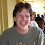## Monday, June 16, 2014

### Ref/info request for an obvious appraoch to GI

Talking about Graphi Isom with some students they came up with the following idea which is certainly not new; however, I couldn't quite find much about it on the web.

ALG(G,H): Let G(0,1) and H(0,1) be the usual adacency matrix using 0 for EDGE NOT THERE and 1 or EDGE IS THERE. Choose n random pairs of numbers (a1,b1), (a2,b2),...,(an,bn) all \le n^2 (happy to change the bound if it will make it work better). For all i see if DET(G(ai,bi))=DET(H(ai,bi)). If there is an i such that they are NOT equal than output NOT ISOM (with complete certainly). If they are ALWAYS equal then output GEE, THOSE GRAPHS ARE PROB ISOMORPHIC.

1. I person who used to work on GI told me that he THINKS there are graphs G, H where the polynomials G(x,y) and H(x,y) are identical yet G and H are not isom. So their are some G,H where the above will definitely fail. Not surprising.
2. Are such graphs rare? Will this work on `almost all pairs of graphs' ? Not clear what that means.
3. If we also checked degree sequences and degrees-of-degrees, would that help? I doubt it since I think that graphs for which this fails are very similar in other ways, but I don't know that.
When I first heard the idea from my students it was new to me. Even so, I knew that it wasn't new and that it there has to be graphs it failes on (item 1 above). How did I know this? If there were no graphs that it failed on then GI would be in R. And if GI was in R, I would know that. And so would you. But this is not a good argument to give to students.

I think its a good idea and it might work for our purposes (which may be a topic of a later blog).

1.Pretty sure we tried this years ago and it fails.

dick lipton

2.It works poorly if the graph contains twin vertices, for one...

However, I have no idea about the general power of the scheme.

3.Frank Harary, The determinant of the adjacency matrix of a graph, SIAM Review, Vol. 4, No. 3. (Jul., 1962), pp. 202-210, It mentions counterexamples with 16 vertices by Bose and others but does not include them, saying that they will be "soon appear in the literature". These are likely strongly regular graphs of some sort. The natural reference is:

Bose, R.C.: Strongly regular graphs, partial geometries and
partially balanced designs. Pacific J. Math., 13 (1963),

but it is not online. In a later paper, Bose discusses a couple of non-isomorphic graphs on 16 vertices that seem to be the likely candidates but I haven't checked them:

(a) Arrange the integers 1 through 16 corresponding to
the vertices of the graph in a 4 x 4 scheme.
1 2 3 4
5 6 7 8
9 10 11 12
13 14 15 16
Then any two vertices are adjacent if and only if the corresponding
integers are in the same row or same column.
(b) Arrange the integers corresponding to the vertices in a
4 x 4 scheme, and impose on it a cyclic Latin square. We thus obtain
1A 2B 3C 4D
5D 6A 7B 8C
9C 10D llA 12B
13B 14C 15D 16A
Two vertices are adjacent if they do not occur in the same row or
in the same column, and do not come together with the same letter
of the cyclic square.

4.Threadjack. Do papers on unsuccessful approaches and attempts get published anymore? They seem useful.

5.1. The graph pair in page 3 of https://orion.math.iastate.edu/butler/PDF/spectra_lecture_1.pdf is a counter example. The determinant in both the cases are zero.

2. GI is easy for most graphs. I would guess that this type of graphs of are also rare.

6.More generally, you can compute and compare the characteristic polynomials  of the two input graphs. However, this graph polynomial is not complete, which means that there are non-isomorphic graphs with the same characteristic polynomial .

 https://en.wikipedia.org/wiki/Characteristic_polynomial

7.I'll admit to not entirely following your notation, Bill, but i'll take the opportunity to point out this paper by Steve Butler which gives methods to generate pairs of non-isomorphic graphs which share the same eigenvalues.

A construction of cospectral graphs for the normalized Laplacian

The paper's results are focused on the normalized Laplacian matrix, but it includes techniques for several common graph matrices